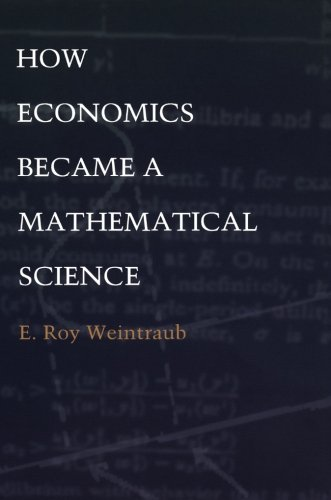# How Economics Became a Mathematical Science (Science and Cultural Theory)Type
Book
Authors
Weintraub ( E. Roy Weintraub )

ISBN 10
0822328712
ISBN 13
9780822328711
Category
EIPE  [ Browse Items ]
Publication Year
2002
Publisher
Pages
328
Tags

Abstract

Description
In How Economics Became a Mathematical Science E. Roy Weintraub traces the history of economics through the prism of the history of mathematics in the twentieth century. As mathematics has evolved, so has the image of mathematics, explains Weintraub, such as ideas about the standards for accepting proof, the meaning of rigor, and the nature of the mathematical enterprise itself. He also shows how economics itself has been shaped by economists’ changing images of mathematics. Whereas others have viewed economics as autonomous, Weintraub presents a different picture, one in which changes in mathematics—both within the body of knowledge that constitutes mathematics and in how it is thought of as a discipline and as a type of knowledge—have been intertwined with the evolution of economic thought. Weintraub begins his account with Cambridge University, the intellectual birthplace of modern economics, and examines specifically Alfred Marshall and the Mathematical Tripos examinations—tests in mathematics that were required of all who wished to study economics at Cambridge. He proceeds to interrogate the idea of a rigorous mathematical economics through the connections between particular mathematical economists and mathematicians in each of the decades of the first half of the twentieth century, and thus describes how the mathematical issues of formalism and axiomatization have shaped economics. Finally, How Economics Became a Mathematical Science reconstructs the career of the economist Sidney Weintraub, whose relationship to mathematics is viewed through his relationships with his mathematician brother, Hal, and his mathematician-economist son, the book’s author. - from Amzon
Biblio Notes

Number of Copies

### Buy online (\$)

#### REVIEWS (0) -

No reviews posted yet.